## Plot basics

All ggplot2 plots begin with a call to ggplot(), supplying default data and aesthetic mappings, specified by aes(). You then add layers, scales, coords and facets with +. To save a plot to disk, use ggsave().

ggplot()
Create a new ggplot
aes()
Construct aesthetic mappings
+(<gg>) %+%
ggsave()
Save a ggplot (or other grid object) with sensible defaults
qplot() quickplot()
Quick plot

## Layers

### Geoms

A layer combines data, aesthetic mapping, a geom (geometric object), a stat (statistical transformation), and a position adjustment. Typically, you will create layers using a geom_ function, overriding the default position and stat if needed.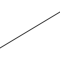geom_abline() geom_hline() geom_vline()
Reference lines: horizontal, vertical, and diagonal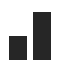geom_bar() geom_col() stat_count()
Bar charts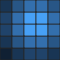geom_bin_2d() stat_bin_2d()
Heatmap of 2d bin counts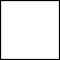geom_blank()
Draw nothing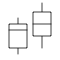geom_boxplot() stat_boxplot()
A box and whiskers plot (in the style of Tukey)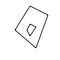geom_contour() geom_contour_filled() stat_contour() stat_contour_filled()
2D contours of a 3D surface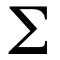geom_count() stat_sum()
Count overlapping points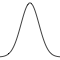geom_density() stat_density()
Smoothed density estimates
geom_density_2d() geom_density_2d_filled() stat_density_2d() stat_density_2d_filled()
Contours of a 2D density estimate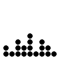geom_dotplot()
Dot plot
geom_errorbarh()
Horizontal error bars
geom_function() stat_function()
Draw a function as a continuous curve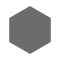geom_hex() stat_bin_hex()
Hexagonal heatmap of 2d bin counts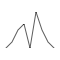geom_freqpoly() geom_histogram() stat_bin()
Histograms and frequency polygons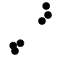geom_jitter()
Jittered points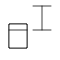geom_crossbar() geom_errorbar() geom_linerange() geom_pointrange()
Vertical intervals: lines, crossbars & errorbars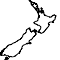geom_map()
Polygons from a reference map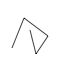geom_path() geom_line() geom_step()
Connect observations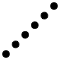geom_point()
Points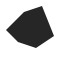geom_polygon()
Polygons
geom_qq_line() stat_qq_line() geom_qq() stat_qq()
A quantile-quantile plot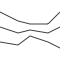geom_quantile() stat_quantile()
Quantile regression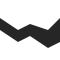geom_ribbon() geom_area() stat_align()
Ribbons and area plots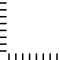geom_rug()
Rug plots in the margins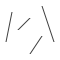geom_segment() geom_curve()
Line segments and curvesgeom_smooth() stat_smooth()
Smoothed conditional means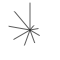geom_spoke()
Line segments parameterised by location, direction and distance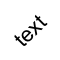geom_label() geom_text()
Text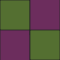geom_raster() geom_rect() geom_tile()
Rectangles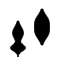geom_violin() stat_ydensity()
Violin plotcoord_sf() geom_sf() geom_sf_label() geom_sf_text() stat_sf()
Visualise sf objects

### Stats

A handful of layers are more easily specified with a stat_ function, drawing attention to the statistical transformation rather than the visual appearance. The computed variables can be mapped using after_stat().

stat_ecdf()
Compute empirical cumulative distribution
stat_ellipse()
Compute normal data ellipses
geom_function() stat_function()
Draw a function as a continuous curve
stat_identity()
Leave data as is
stat_summary_2d() stat_summary_hex()
Bin and summarise in 2d (rectangle & hexagons)
stat_summary_bin() stat_summary()
Summarise y values at unique/binned x
stat_unique()
Remove duplicates
stat_sf_coordinates()
Extract coordinates from 'sf' objects
after_stat() after_scale() stage()
Control aesthetic evaluation

All layers have a position adjustment that resolves overlapping geoms. Override the default by using the position argument to the geom_ or stat_ function.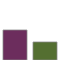position_dodge() position_dodge2()
Dodge overlapping objects side-to-side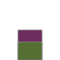position_identity()position_jitter()
Jitter points to avoid overplotting
position_jitterdodge()
Simultaneously dodge and jitter
position_nudge()
Nudge points a fixed distance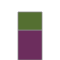position_stack() position_fill()
Stack overlapping objects on top of each another

### Annotations

Annotations are a special type of layer that don’t inherit global settings from the plot. They are used to add fixed reference data to plots.geom_abline() geom_hline() geom_vline()
Reference lines: horizontal, vertical, and diagonal
annotate()
Create an annotation layer
annotation_custom()
Annotation: Custom grob
annotation_logticks()
Annotation: log tick marks
annotation_map()
Annotation: a map
annotation_raster()
Annotation: high-performance rectangular tiling
borders()
Create a layer of map borders

## Aesthetics

The following help topics give a broad overview of some of the ways you can use each aesthetic.

aes_colour_fill_alpha colour color fill
Colour related aesthetics: colour, fill, and alpha
aes_group_order group
Aesthetics: grouping
aes_linetype_size_shape linetype size shape
Differentiation related aesthetics: linetype, size, shape
aes_position x y xmin xmax ymin ymax xend yend
Position related aesthetics: x, y, xmin, xmax, ymin, ymax, xend, yend

## Scales

Scales control the details of how data values are translated to visual properties. Override the default scales to tweak details like the axis labels or legend keys, or to use a completely different translation from data to aesthetic. labs() and lims() are convenient helpers for the most common adjustments to the labels and limits.

labs() xlab() ylab() ggtitle()
Modify axis, legend, and plot labels
lims() xlim() ylim()
Set scale limits
expand_limits()
Expand the plot limits, using data
expansion() expand_scale()
Generate expansion vector for scales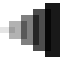scale_alpha() scale_alpha_continuous() scale_alpha_binned() scale_alpha_discrete() scale_alpha_ordinal()
Alpha transparency scales
scale_x_binned() scale_y_binned()
Positional scales for binning continuous data (x & y)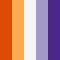scale_colour_brewer() scale_fill_brewer() scale_colour_distiller() scale_fill_distiller() scale_colour_fermenter() scale_fill_fermenter()
Sequential, diverging and qualitative colour scales from ColorBrewer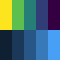scale_colour_continuous() scale_fill_continuous() scale_colour_binned() scale_fill_binned()
Continuous and binned colour scales
scale_colour_discrete() scale_fill_discrete()
Discrete colour scales
scale_x_continuous() scale_y_continuous() scale_x_log10() scale_y_log10() scale_x_reverse() scale_y_reverse() scale_x_sqrt() scale_y_sqrt()
Position scales for continuous data (x & y)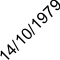scale_x_date() scale_y_date() scale_x_datetime() scale_y_datetime() scale_x_time() scale_y_time()
Position scales for date/time data
scale_x_discrete() scale_y_discrete()
Position scales for discrete data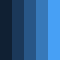scale_colour_gradient() scale_fill_gradient() scale_colour_gradient2() scale_fill_gradient2() scale_colour_gradientn() scale_fill_gradientn()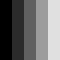scale_colour_grey() scale_fill_grey()
Sequential grey colour scales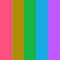scale_colour_hue() scale_fill_hue()
Evenly spaced colours for discrete data
scale_colour_identity() scale_fill_identity() scale_shape_identity() scale_linetype_identity() scale_linewidth_identity() scale_alpha_identity() scale_size_identity() scale_discrete_identity() scale_continuous_identity()
Use values without scaling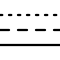scale_linetype() scale_linetype_binned() scale_linetype_continuous() scale_linetype_discrete()
Scale for line patterns
scale_linewidth() scale_linewidth_binned()
Scales for line width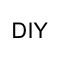scale_colour_manual() scale_fill_manual() scale_size_manual() scale_shape_manual() scale_linetype_manual() scale_linewidth_manual() scale_alpha_manual() scale_discrete_manual()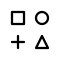scale_shape() scale_shape_binned()
Scales for shapes, aka glyphs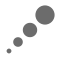scale_size() scale_radius() scale_size_binned() scale_size_area() scale_size_binned_area()
scale_colour_steps() scale_colour_steps2() scale_colour_stepsn() scale_fill_steps() scale_fill_steps2() scale_fill_stepsn()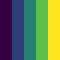scale_colour_viridis_d() scale_fill_viridis_d() scale_colour_viridis_c() scale_fill_viridis_c() scale_colour_viridis_b() scale_fill_viridis_b()
Viridis colour scales from viridisLite
get_alt_text()
Extract alt text from a plot

## Guides: axes and legends

The guides (the axes and legends) help readers interpret your plots. Guides are mostly controlled via the scale (e.g. with the limits, breaks, and labels arguments), but sometimes you will need additional control over guide appearance. Use guides() or the guide argument to individual scales along with guide_*() functions.

draw_key_point() draw_key_abline() draw_key_rect() draw_key_polygon() draw_key_blank() draw_key_boxplot() draw_key_crossbar() draw_key_path() draw_key_vpath() draw_key_dotplot() draw_key_linerange() draw_key_pointrange() draw_key_smooth() draw_key_text() draw_key_label() draw_key_vline() draw_key_timeseries()
Key glyphs for legends
guide_colourbar() guide_colorbar()
Continuous colour bar guide
guide_legend()
Legend guide
guide_axis()
Axis guide
guide_bins()
A binned version of guide_legend
guide_coloursteps() guide_colorsteps()
Discretized colourbar guide
guide_none()
Empty guide
guides()
Set guides for each scale
sec_axis() dup_axis() derive()
Specify a secondary axis

## Facetting

Facetting generates small multiples, each displaying a different subset of the data. Facets are an alternative to aesthetics for displaying additional discrete variables.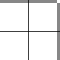facet_grid()
Lay out panels in a grid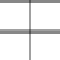facet_wrap()
Wrap a 1d ribbon of panels into 2d
vars()
Quote faceting variables

### Labels

These functions provide a flexible toolkit for controlling the display of the “strip” labels on facets.

labeller()
Construct labelling specification
label_value() label_both() label_context() label_parsed() label_wrap_gen()
Useful labeller functions
label_bquote()
Label with mathematical expressions

## Coordinate systems

The coordinate system determines how the x and y aesthetics combine to position elements in the plot. The default coordinate system is Cartesian (coord_cartesian()), which can be tweaked with coord_map(), coord_fixed(), coord_flip(), and coord_trans(), or completely replaced with coord_polar().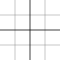coord_cartesian()
Cartesian coordinates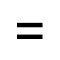coord_fixed()
Cartesian coordinates with fixed "aspect ratio"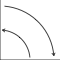coord_flip()
Cartesian coordinates with x and y flippedcoord_map() coord_quickmap()
Map projections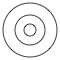coord_polar()
Polar coordinates
coord_trans()
Transformed Cartesian coordinate system

## Themes

Themes control the display of all non-data elements of the plot. You can override all settings with a complete theme like theme_bw(), or choose to tweak individual settings by using theme() and the element_ functions. Use theme_set() to modify the active theme, affecting all future plots.

theme()
Modify components of a theme
theme_grey() theme_gray() theme_bw() theme_linedraw() theme_light() theme_dark() theme_minimal() theme_classic() theme_void() theme_test()
Complete themes
theme_get() theme_set() theme_update() theme_replace() %+replace%
Get, set, and modify the active theme
margin() element_blank() element_rect() element_line() element_text() rel()
Theme elements

## Programming with ggplot2

These functions provides tools to help you program with ggplot2, creating functions and for-loops that generate plots for you.

aes_() aes_string() aes_q()
Define aesthetic mappings programmatically
print(<ggplot>) plot(<ggplot>)
Explicitly draw plot

## Extending ggplot2

To create your own geoms, stats, scales, and facets, you’ll need to learn a bit about the object oriented system that ggplot2 uses. Start by reading vignette("extending-ggplot2") then consult these functions for more details.

ggproto() ggproto_parent() is.ggproto()
Create a new ggproto object
print(<ggproto>) format(<ggproto>)
Format or print a ggproto object

## Vector helpers

ggplot2 also provides a handful of helpers that are useful for creating visualisations.

cut_interval() cut_number() cut_width()
Discretise numeric data into categorical
mean_cl_boot() mean_cl_normal() mean_sdl() median_hilow()
A selection of summary functions from Hmisc
mean_se()
Calculate mean and standard error of the mean
resolution()
Compute the "resolution" of a numeric vector

## Data

ggplot2 comes with a selection of built-in datasets that are used in examples to illustrate various visualisation challenges.

diamonds
Prices of over 50,000 round cut diamonds
economics economics_long
US economic time series
faithfuld
2d density estimate of Old Faithful data
midwest
Midwest demographics
mpg
Fuel economy data from 1999 to 2008 for 38 popular models of cars
msleep
An updated and expanded version of the mammals sleep dataset
presidential
Terms of 12 presidents from Eisenhower to Trump
seals
Vector field of seal movements
txhousing
Housing sales in TX
luv_colours
colors() in Luv space

## Autoplot and fortify

autoplot() is an extension mechanism for ggplot2: it provides a way for package authors to add methods that work like the base plot() function, generating useful default plots with little user interaction. fortify() turns objects into tidy data frames: it has largely been superseded by the broom package.

autoplot()
Create a complete ggplot appropriate to a particular data type
autolayer()
Create a ggplot layer appropriate to a particular data type
fortify()
Fortify a model with data.
map_data()
Create a data frame of map data